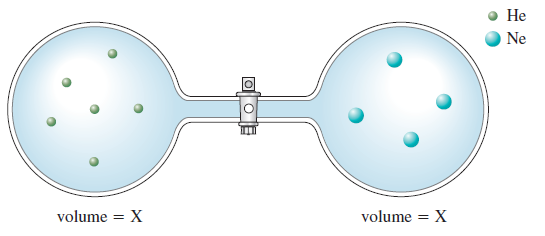# Problem: Consider the flasks in the following diagrams.c. Solve for the final pressure in terms of the original pressures of helium and neon. Assume temperature is constant.

⚠️Our tutors found the solution shown to be helpful for the problem you're searching for. We don't have the exact solution yet.

###### Problem Details

Consider the flasks in the following diagrams.c. Solve for the final pressure in terms of the original pressures of helium and neon. Assume temperature is constant.